# 梯度下降算法原理讲解——机器学习

### 博文目录

#### 2. 梯度下降算法

##### 2.1 场景假设##### 2.2 梯度下降

###### 2.2.1 微分

• 函数图像中，某点的切线的斜率
• 函数的变化率
几个微分的例子：

1.单变量的微分，函数只有一个变量时

d ( x 2 ) d x = 2 x \frac{d(x^2)}{dx}=2x

d ( − 2 y 5 ) d y = − 10 y 4 \frac{d(-2y^5)}{dy}=-10y^4

d ( 5 − θ ) 2 d θ = − 2 ( 5 − θ ) \frac{d(5-\theta )^2}{d\theta}=-2(5-\theta)

2.多变量的微分，当函数有多个变量的时候，即分别对每个变量进行求微分

∂ ∂ x ( x 2 y 2 ) = 2 x y 2 \frac{\partial}{\partial x}(x^2y^2) = 2xy^2

∂ ∂ y ( − 2 y 5 + z 2 ) = − 10 y 4 \frac{\partial}{\partial y}(-2y^5+z^2) = -10y^4

∂ ∂ θ 2 ( 5 θ 1 + 2 θ 2 − 12 θ 3 ) = 2 \frac{\partial}{\partial \theta_{2}}(5\theta_{1} + 2\theta_{2} - 12\theta_{3}) = 2

∂ ∂ θ 2 ( 0.55 − ( 5 θ 1 + 2 θ 2 − 12 θ 3 ) ) = − 2 \frac{\partial}{\partial \theta_{2}}(0.55 - (5\theta_{1} + 2\theta_{2} - 12\theta_{3})) = -2

###### 2.2.2 梯度

J ( Θ ) = 0.55 − ( 5 θ 1 + 2 θ 2 − 12 θ 3 ) J(\Theta ) = 0.55 - (5\theta_{1} + 2\theta_{2} - 12\theta_{3})

▽ J ( Θ ) = < ∂ J ∂ θ 1 , ∂ J ∂ θ 2 , ∂ J ∂ θ 3 > = ( − 5 , − 2 , 12 ) \triangledown J(\Theta ) = \left < \frac{\partial J}{\partial \theta_{1}}, \frac{\partial J}{\partial \theta_{2}},\frac{\partial J}{\partial \theta_{3}} \right > =(-5,-2,12)

• 在单变量的函数中，梯度其实就是函数的微分，代表着函数在某个给定点的切线的斜率
• 在多变量函数中，梯度是一个向量，向量有方向，梯度的方向就指出了函数在给定点的上升最快的方向

##### 2.3 数学解释

Θ 1 = Θ 0 + α ▽ J ( Θ ) → e v a l u a t e d a t Θ 0 {\color{Red} \Theta^1} = {\color{Blue} \Theta^0} + {\color{Green} \alpha} {\color{Purple} \triangledown J(\Theta)}\rightarrow evaluated at \Theta^0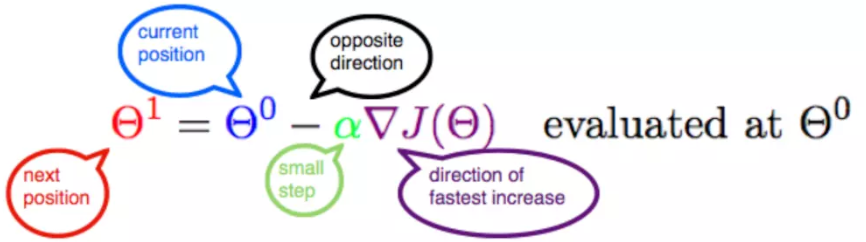###### 2.3.1 α

α在梯度下降算法中被称作为学习率或者步长，意味着我们可以通过α来控制每一步走的距离，以保证不要步子跨的太大扯着蛋，哈哈，其实就是不要走太快，错过了最低点。同时也要保证不要走的太慢，导致太阳下山了，还没有走到山下。所以α的选择在梯度下降法中往往是很重要的！α不能太大也不能太小，太小的话，可能导致迟迟走不到最低点，太大的话，会导致错过最低点！

#### 3. 实例

##### 3.1 单变量函数的梯度下降

J ( θ ) = θ 2 J(\theta) = \theta^2

J ′ ( θ ) = 2 θ J'(\theta) = 2\theta

θ 0 = 1 \theta^0 = 1

α = 0.4 \alpha = 0.4

Θ 1 = Θ 0 + α ▽ J ( Θ ) → e v a l u a t e d a t Θ 0 {\color{Red} \Theta^1} = {\color{Blue} \Theta^0} + {\color{Green} \alpha} {\color{Purple} \triangledown J(\Theta)}\rightarrow evaluated at \Theta^0

θ 0 = 1 \theta^0 = 1

θ 1 = θ 0 − α ∗ J ′ ( θ 0 ) = 1 − 0.4 ∗ 2 = 0.2 \theta^1 = \theta^0 - \alpha*J'(\theta^0)=1 - 0.4*2 = 0.2

θ 2 = θ 1 − α ∗ J ′ ( θ 1 ) = 0.2 − 0.4 ∗ 0.4 = 0.04 \theta^2 = \theta^1 - \alpha*J'(\theta^1)= 0.2 - 0.4*0.4=0.04

θ 3 = 0.008 \theta^3 = 0.008

θ 4 = 0.0016 \theta^4 = 0.0016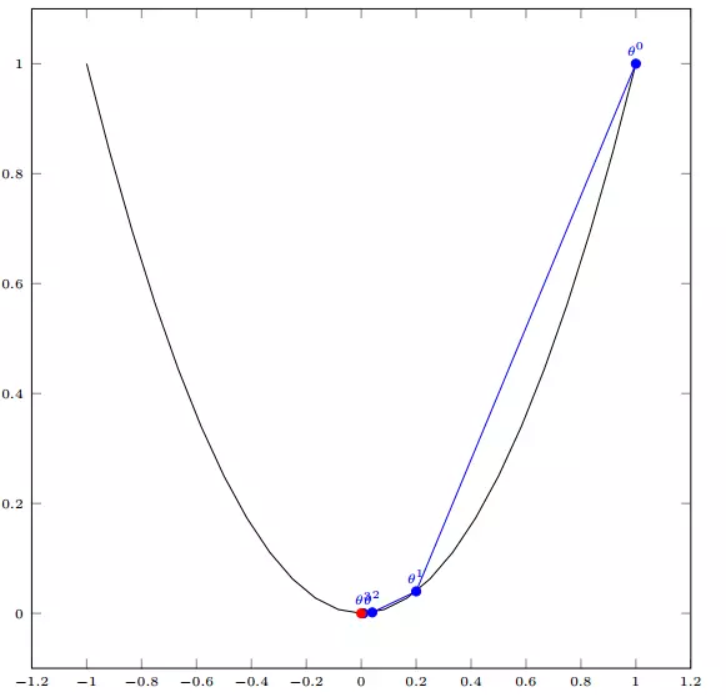##### 3.2 多变量函数的梯度下降

J ( Θ ) = θ 1 2 + θ 2 2 J(\Theta) = \theta_{1}^2 + \theta_{2}^2

Θ 0 = ( 1 , 3 ) \Theta^0 = (1, 3)

α = 0.1 \alpha = 0.1

▽ J ( Θ ) = < 2 θ 1 , 2 θ 2 > \triangledown J(\Theta ) = \left < 2\theta_{1},2\theta_{2} \right >

Θ 0 = ( 1 , 3 ) \Theta^0 = (1, 3)

Θ 1 = Θ 0 − α ▽ J ( Θ ) = ( 1 , 3 ) − 0.1 ∗ ( 2 , 6 ) = ( 0.8 , 2.4 ) \Theta^1 = \Theta^0 - \alpha\triangledown J(\Theta ) = (1,3) - 0.1*(2, 6)=(0.8, 2.4)

Θ 2 = ( 0.8 , 2.4 ) − 0.1 ∗ ( 1.6 , 4.8 ) = ( 0.64 , 1.92 ) \Theta^2 = (0.8, 2.4) - 0.1*(1.6, 4.8)=(0.64, 1.92)

Θ 3 = ( 0.5124 , 1.536 ) \Theta^3 =(0.5124, 1.536)

Θ 4 = ( 0.4096 , 1.228800000000001 ) \Theta^4 =(0.4096, 1.228800000000001)
⋮ \vdots
Θ 10 = ( 0.1073741824000003 , 0.32212254720000005 ) \Theta^{10} =(0.1073741824000003, 0.32212254720000005)
⋮ \vdots
Θ 50 = ( 1.141798154164342 e − 05 , 3.42539442494306 e − 05 ) \Theta^{50} =(1.141798154164342e^{-05}, 3.42539442494306e^{-05})
⋮ \vdots
Θ 100 = ( 1.6296287810675902 e − 10 , 4.8888886343202771 e − 10 ) \Theta^{100} =(1.6296287810675902e^{-10}, 4.8888886343202771e^{-10})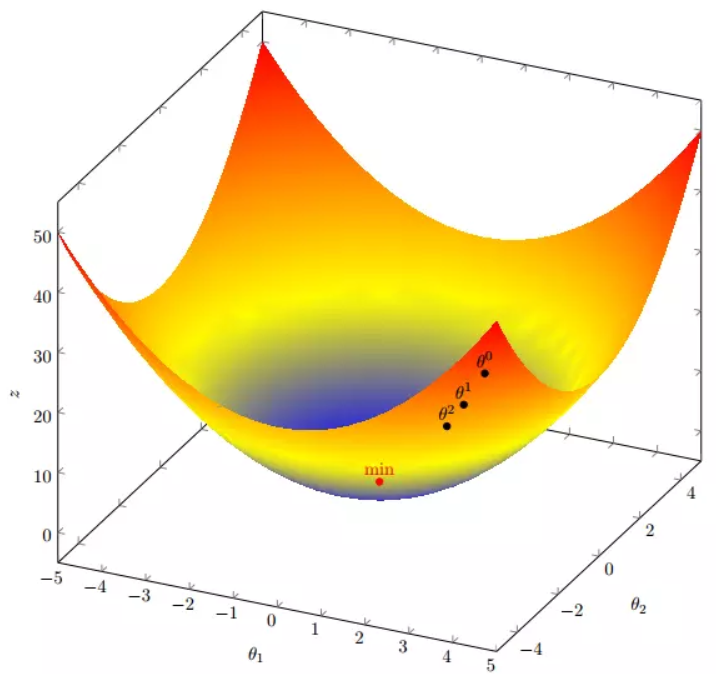#### 4. 代码实现

##### 4. 1 场景分析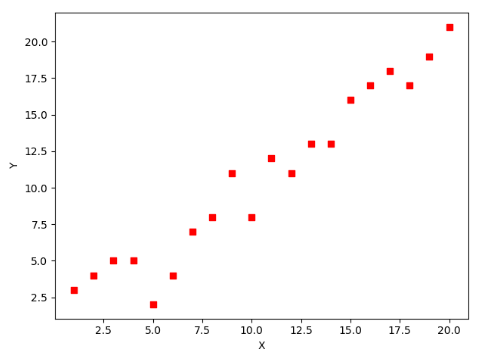J ( Θ ) = 1 2 m ∑ i = 1 m ( h θ ( x ( i ) ) − y ( i ) ) 2 J(\Theta) = \frac{1}{2m}\sum_{i=1}^{m}(h_{\theta}(x^{(i)})-y^{(i)})^2

• m是数据集中数据点的个数，也就是样本数
• ½是一个常量，这样是为了在求梯度的时候，二次方乘下来的2就和这里的½抵消了，自然就没有多余的常数系数，方便后续的计算，同时对结果不会有影响
• y 是数据集中每个点的真实y坐标的值，也就是类标签
• h 是我们的预测函数（假设函数），根据每一个输入x，根据Θ 计算得到预测的y值，即

h Θ ( x ( i ) ) = Θ 0 + Θ 1 x 1 ( i ) h_{\Theta}(x^{(i)}) = \Theta_{0} + \Theta_{1}x_{1}^{(i)}

▽ J ( Θ ) = < δ J δ Θ 0 , δ J δ Θ 1 > \triangledown J(\Theta ) = \left < \frac{\delta J}{\delta \Theta_{0}}, \frac{\delta J}{\delta \Theta_{1}} \right >

δ J δ Θ 0 = 1 m ∑ i = 1 m ( h Θ ( x ( i ) ) − y ( i ) ) \frac{\delta J}{\delta \Theta_{0}} = \frac{1}{m}\sum_{i=1}^{m}(h_{\Theta}(x^{(i)})-y^{(i)})

δ J δ Θ 1 = 1 m ∑ i = 1 m ( h Θ ( x ( i ) ) − y ( i ) ) x 1 ( i ) \frac{\delta J}{\delta \Theta_{1}} = \frac{1}{m}\sum_{i=1}^{m}(h_{\Theta}(x^{(i)})-y^{(i)})x_{1}^{(i)}

h Θ ( x ( i ) ) = Θ 0 + Θ 1 x ( i ) h_{\Theta}(x^{(i)}) = \Theta_{0} + \Theta_{1}x^{(i)}

( x 1 ( i ) , y ( i ) ) → ( x 0 ( i ) , x 1 ( i ) , y ( i ) ) w i t h x 0 ( i ) = 1 ∀ i (x_{1}^{(i)},y^{(i)})\rightarrow (x_{0}^{(i)},x_{1}^{(i)},y^{(i)}) with x_{0}^{(i)} = 1 \forall _{i}

J ( Θ ) = 1 2 m ( X Θ − y ⃗ ) T ( X Θ − y ⃗ ) J(\Theta) = \frac{1}{2m}(X\Theta - \vec{y})^{T}(X\Theta - \vec{y})

▽ J ( Θ ) = 1 m X T ( X Θ − y ⃗ ) ) \triangledown J(\Theta) = \frac{1}{m}X^{T}(X\Theta - \vec{y}))

##### 4. 2 代码

#!/usr/bin/env python3
# -*- coding: utf-8 -*-
# @Time    : 2019/1/21 21:06
# @Author  : Arrow and Bullet
# @Software: PyCharm
# @Blog    ：https://blog.csdn.net/qq_41800366

from numpy import *

# 数据集大小 即20个数据点
m = 20
# x的坐标以及对应的矩阵
X0 = ones((m, 1))  # 生成一个m行1列的向量，也就是x0，全是1
X1 = arange(1, m+1).reshape(m, 1)  # 生成一个m行1列的向量，也就是x1，从1到m
X = hstack((X0, X1))  # 按照列堆叠形成数组，其实就是样本数据
# 对应的y坐标
y = np.array([
3, 4, 5, 5, 2, 4, 7, 8, 11, 8, 12,
11, 13, 13, 16, 17, 18, 17, 19, 21
]).reshape(m, 1)
# 学习率
alpha = 0.01


# 定义代价函数
def cost_function(theta, X, Y):
diff = dot(X, theta) - Y  # dot() 数组需要像矩阵那样相乘，就需要用到dot()
return (1/(2*m)) * dot(diff.transpose(), diff)

# 定义代价函数对应的梯度函数
diff = dot(X, theta) - Y
return (1/m) * dot(X.transpose(), diff)


# 梯度下降迭代
theta = array([1, 1]).reshape(2, 1)
theta = theta - alpha * gradient
return theta

print('optimal:', optimal)
print('cost function:', cost_function(optimal, X, Y))


print('optimal:', optimal)  # 结果 [[0.51583286][0.96992163]]
print('cost function:', cost_function(optimal, X, Y))  # 1.014962406233101


# 根据数据画出对应的图像
def plot(X, Y, theta):
import matplotlib.pyplot as plt
ax = plt.subplot(111)  # 这是我改的
ax.scatter(X, Y, s=30, c="red", marker="s")
plt.xlabel("X")
plt.ylabel("Y")
x = arange(0, 21, 0.2)  # x的范围
y = theta + theta*x
ax.plot(x, y)
plt.show()

plot(X1, Y, optimal)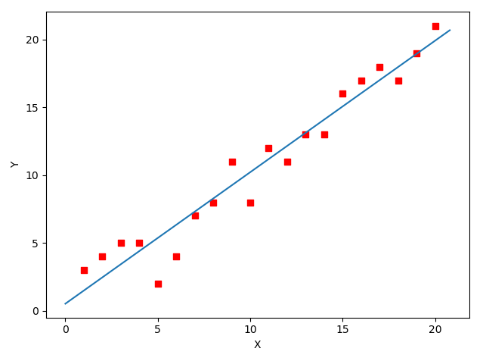#!/usr/bin/env python3
# -*- coding: utf-8 -*-
# @Time    : 2019/1/21 21:06
# @Author  : Arrow and Bullet
# @Software: PyCharm
# @Blog    ：https://blog.csdn.net/qq_41800366

from numpy import *

# 数据集大小 即20个数据点
m = 20
# x的坐标以及对应的矩阵
X0 = ones((m, 1))  # 生成一个m行1列的向量，也就是x0，全是1
X1 = arange(1, m+1).reshape(m, 1)  # 生成一个m行1列的向量，也就是x1，从1到m
X = hstack((X0, X1))  # 按照列堆叠形成数组，其实就是样本数据
# 对应的y坐标
Y = array([
3, 4, 5, 5, 2, 4, 7, 8, 11, 8, 12,
11, 13, 13, 16, 17, 18, 17, 19, 21
]).reshape(m, 1)
# 学习率
alpha = 0.01

# 定义代价函数
def cost_function(theta, X, Y):
diff = dot(X, theta) - Y  # dot() 数组需要像矩阵那样相乘，就需要用到dot()
return (1/(2*m)) * dot(diff.transpose(), diff)

# 定义代价函数对应的梯度函数
diff = dot(X, theta) - Y
return (1/m) * dot(X.transpose(), diff)

# 梯度下降迭代
theta = array([1, 1]).reshape(2, 1)
theta = theta - alpha * gradient
return theta

print('optimal:', optimal)
print('cost function:', cost_function(optimal, X, Y))

# 根据数据画出对应的图像
def plot(X, Y, theta):
import matplotlib.pyplot as plt
ax = plt.subplot(111)  # 这是我改的
ax.scatter(X, Y, s=30, c="red", marker="s")
plt.xlabel("X")
plt.ylabel("Y")
x = arange(0, 21, 0.2)  # x的范围
y = theta + theta*x
ax.plot(x, y)
plt.show()

plot(X1, Y, optimal)


#### 5. 小结

07-057万+11-033万+
12-0516万+
10-17673
11-299万+
04-191万+
10-25
10-25518
10-157303
01-071万+
12-146万+
08-02471
01-248588
11-295913
07-038210
04-143万+
04-26510
02-265471
©️2020 CSDN 皮肤主题: Age of Ai 设计师:meimeiellie